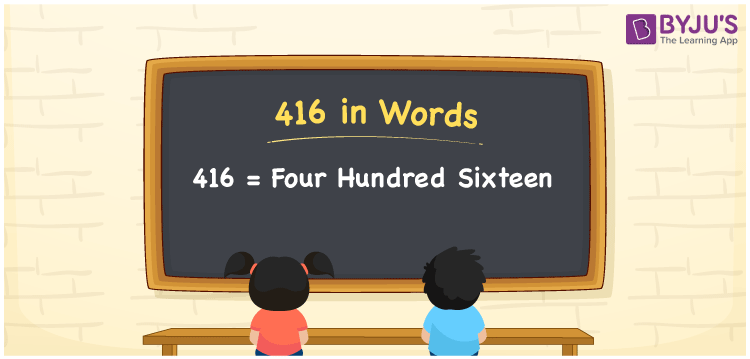# 416 in words

416 in words is written as Four Hundred and Sixteen. 416 represents the count or value. The article on Counting Numbers can give you an idea about count or counting. The number 416 is used in expressions that relate to money, distance, length, year and others. Let us consider an example for 416. ”The walk from my home to office is hardly Four Hundred and Sixteen meters”.

 416 in words Four Hundred and Sixteen Four Hundred and Sixteen in Numbers 416

## 416 in English Words## How to Write 416 in Words?

We can convert 416 to words using a place value chart. The number 416 has 3 digits, so let’s make a chart that shows the place value up to 3 digits.

 Hundreds Tens Ones 4 1 6

Thus, we can write the expanded form as:

= 4 × Hundred + 1 × Ten + 6 × One

= 4 × 100 + 1 × 10 + 6 × 1

= 416

= Four Hundred and Sixteen.

416 is the natural number that is succeeded by 415 and preceded by 417.

416 in words – Four Hundred and Sixteen.

Is 416 an odd number? – No.

Is 416 an even number? – Yes.

Is 416 a perfect square number? – No.

Is 416 a perfect cube number? – No.

Is 416 a prime number? – No.

Is 416 a composite number? – Yes.

## Solved Example

1. Write the number 416 in expanded form

Solution: 4 × 100 + 1 × 10 + 6 × 1

We can write 416 = 400 + 10 + 6

= 4 × 100 + 1 × 10 + 6 × 1

## Frequently Asked Questions on 416 in words

Q1

### How to write 416 in words?

416 in words is written as Four Hundred and Sixteen.
Q2

### Is 416 a perfect square number?

No. 416 is not a perfect square number.
Q3

### Is 416 a prime number?

No. 416 is not a prime number.